# Non linear Regression examples – ML

Non-Linear regression is a type of polynomial regression. It is a method to model a non-linear relationship between the dependent and independent variables. It is used in place when the data shows a curvy trend, and linear regression would not produce very accurate results when compared to non-linear regression. This is because in linear regression it is pre-assumed that the data is linear.

Code:

 `import` `numpy as np ` `import` `pandas as pd ` ` `  `# downloading dataset  ` `! wget ``-``nv ``-``O china_gdp.csv https:``/``/``s3``-``api.us``-``geo.objectstorage.softlayer.net``/` `                    ``cf``-``courses``-``data``/``CognitiveClass``/``ML0101ENv3``/``labs``/``china_gdp.csv ` `     `  `df ``=` `pd.read_csv(``"china_gdp.csv"``) ` ` `  `def` `sigmoid(x, Beta_1, Beta_2): ` `     ``y ``=` `1` `/` `(``1` `+` `np.exp(``-``Beta_1``*``(x``-``Beta_2))) ` `     ``return` `y ` `     `  `beta_1 ``=` `0.10` `beta_2 ``=` `1990.0` ` `  `# logistic function ` `Y_pred ``=` `sigmoid(x_data, beta_1, beta_2) ` ` `  `# plot initial prediction against datapoints ` `plt.plot(x_data, Y_pred ``*` `15000000000000.``) ` `plt.plot(x_data, y_data, ``'ro'``)     `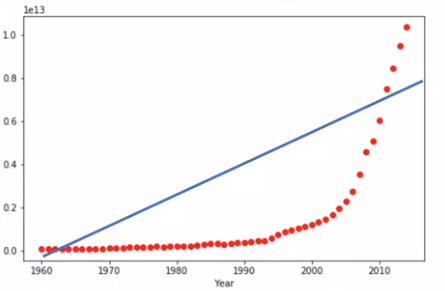The scatter plot shows the relationship between GDP and time of a country, but the relationship is not linear. Instead after 2005 the line starts to become curve and does not follow a linear straight path. In such cases, a special estimation method is required called the non-linear regression.

Code:

 `import` `numpy as np ` `import` `pandas as pd ` ` `  `# downloading dataset  ` `! wget ``-``nv ``-``O china_gdp.csv https:``/``/``s3``-``api.us``-``geo.objectstorage.softlayer.net``/`  `         ``cf``-``courses``-``data ``/` `CognitiveClass ``/` `ML0101ENv3 ``/` `labs ``/` `china_gdp.csv ` `     `  `df ``=` `pd.read_csv(``"china_gdp.csv"``) ` ` `  `def` `sigmoid(x, Beta_1, Beta_2): ` `     ``y ``=` `1` `/` `(``1` `+` `np.exp(``-``Beta_1``*``(x``-``Beta_2))) ` `     ``return` `y ` `      `  `x ``=` `np.linspace(``1960``, ``2015``, ``55``) ` `x ``=` `x ``/` `max``(x) ` `y ``=` `sigmoid(x, ``*``popt) ` ` `  `plt.figure(figsize ``=``(``8``, ``5``)) ` `plt.plot(xdata, ydata, ``'ro'``, label ``=``'data'``) ` `plt.plot(x, y, linewidth ``=` `3.0``, label ``=``'fit'``) ` `plt.legend(loc ``=``'best'``) ` `plt.ylabel(``'GDP'``) ` `plt.xlabel(``'Year'``) ` `plt.show() `

Output: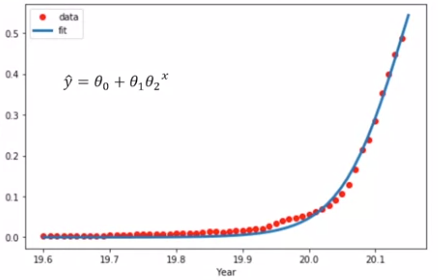There are many different regressions that exists and can be used to fit whatever the dataset looks like such as quadratic, cubic regression, and so on to infinite degrees according to our requirement.

Code:

 `import` `numpy as np ` `import` `matplotlib.pyplot as plt ``%` `matplotlib inline ` ` `  `x ``=` `np.arange(``-``5.0``, ``5.0``, ``0.1``) ` ` `  `## You can adjust the slope and intercept to verify the changes in the graph ` `y ``=` `2``*``(x) ``+` `3` `y_noise ``=` `2` `*` `np.random.normal(size ``=` `x.size) ` `ydata ``=` `y ``+` `y_noise ` `# plt.figure(figsize =(8, 6)) ` `plt.plot(x, ydata,  ``'bo'``) ` `plt.plot(x, y, ``'r'``)  ` `plt.ylabel(``'Dependent Variable'``) ` `plt.xlabel(``'Indepdendent Variable'``) ` `plt.show()`

Output: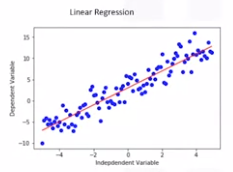Code:

 `import` `numpy as np ` `import` `matplotlib.pyplot as plt ``%` `matplotlib inline ` ` `  `x ``=` `np.arange(``-``5.0``, ``5.0``, ``0.1``) ` ` `  `## You can adjust the slope and intercept to verify the changes in the graph ` ` `  `y ``=` `np.power(x, ``2``) ` `y_noise ``=` `2` `*` `np.random.normal(size ``=` `x.size) ` `ydata ``=` `y ``+` `y_noise ` `plt.plot(x, ydata,  ``'bo'``) ` `plt.plot(x, y, ``'r'``)  ` `plt.ylabel(``'Dependent Variable'``) ` `plt.xlabel(``'Indepdendent Variable'``) ` `plt.show()`

Output: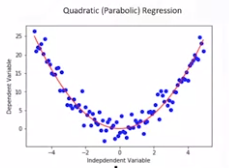Code:

 `import` `numpy as np ` `import` `matplotlib.pyplot as plt ``%` `matplotlib inline ` ` `  `x ``=` `np.arange(``-``5.0``, ``5.0``, ``0.1``) ` ` `  `## You can adjust the slope and intercept to verify the changes in the graph ` `y ``=` `1``*``(x``*``*``3``) ``+` `1``*``(x``*``*``2``) ``+` `1` `*` `x ``+` `3` `y_noise ``=` `20` `*` `np.random.normal(size ``=` `x.size) ` `ydata ``=` `y ``+` `y_noise ` `plt.plot(x, ydata,  ``'bo'``) ` `plt.plot(x, y, ``'r'``)  ` `plt.ylabel(``'Dependent Variable'``) ` `plt.xlabel(``'Indepdendent Variable'``) ` `plt.show() `

Output: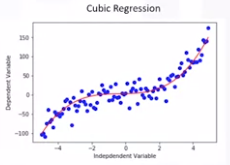Cubic Regression

We can call all of these polynomial regression, where the relationship between the independent variable X and the dependent variable Y is modeled as an Nth degree polynomial in X.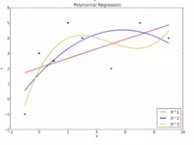Polynomial Regression

For a model to be considered non-linear, Y hat must be a non-linear function of the parameters Theta, not necessarily the features X. When it comes to non-linear equation, it can be the shape of exponential, logarithmic, and logistic, or many other types.
Output: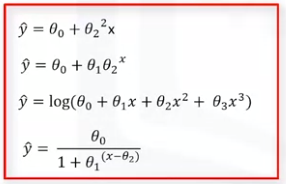As you can see in all of these equations, the change of Y hat depends on changes in the parameters Theta, not necessarily on X only. That is, in non-linear regression, a model is non-linear by parameters.

My Personal Notes arrow_drop_upCheck out this Author's contributed articles.

If you like GeeksforGeeks and would like to contribute, you can also write an article using contribute.geeksforgeeks.org or mail your article to contribute@geeksforgeeks.org. See your article appearing on the GeeksforGeeks main page and help other Geeks.

Please Improve this article if you find anything incorrect by clicking on the "Improve Article" button below.

Article Tags :
Practice Tags :

1

Please write to us at contribute@geeksforgeeks.org to report any issue with the above content.